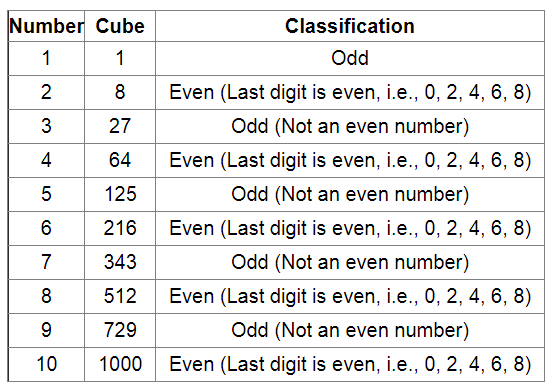# Write the cubes of all natural numbers between 1 and 10 and verify the following statements:`
Question:

Write the cubes of all natural numbers between 1 and 10 and verify the following statements:

(i) Cubes of all odd natural numbers are odd.

(ii) Cubes of all even natural numbers are even.

Solution:

The cubes of natural numbers between 1 and 10 are listed and classified in the following table.

We can classify all natural numbers as even or odd number; therefore, to check whether the cube of a natural number is even or odd, it is sufficient to check its divisibility by 2.

If the number is divisible by 2, it is an even number, otherwise it will an odd number.

(i) From the above table, it is evident that cubes of all odd natural numbers are odd.

(ii) From the above table, it is evident that cubes of all even natural numbers are even.